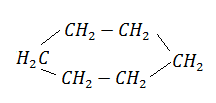NEET Questions Solved

In the following reaction the catalyst used is:$\to$${\mathrm{C}}_{6}{\mathrm{H}}_{6}$ + 3${\mathrm{H}}_{2}$

(a) ${\mathrm{Cr}}_{2}{\mathrm{O}}_{3}$                                      (b) $A{l}_{2}{\mathrm{O}}_{3}$

(c) Zn dust                                    (d) ${\mathrm{Cr}}_{2}{\mathrm{O}}_{3}$ and $A{l}_{2}{\mathrm{O}}_{3}$

(d) Dehydration of cycloalkane is made in presence of ${\mathrm{Cr}}_{2}{\mathrm{O}}_{3}$/$A{l}_{2}{\mathrm{O}}_{3}$.

Difficulty Level:

• 14%
• 11%
• 25%
• 52%